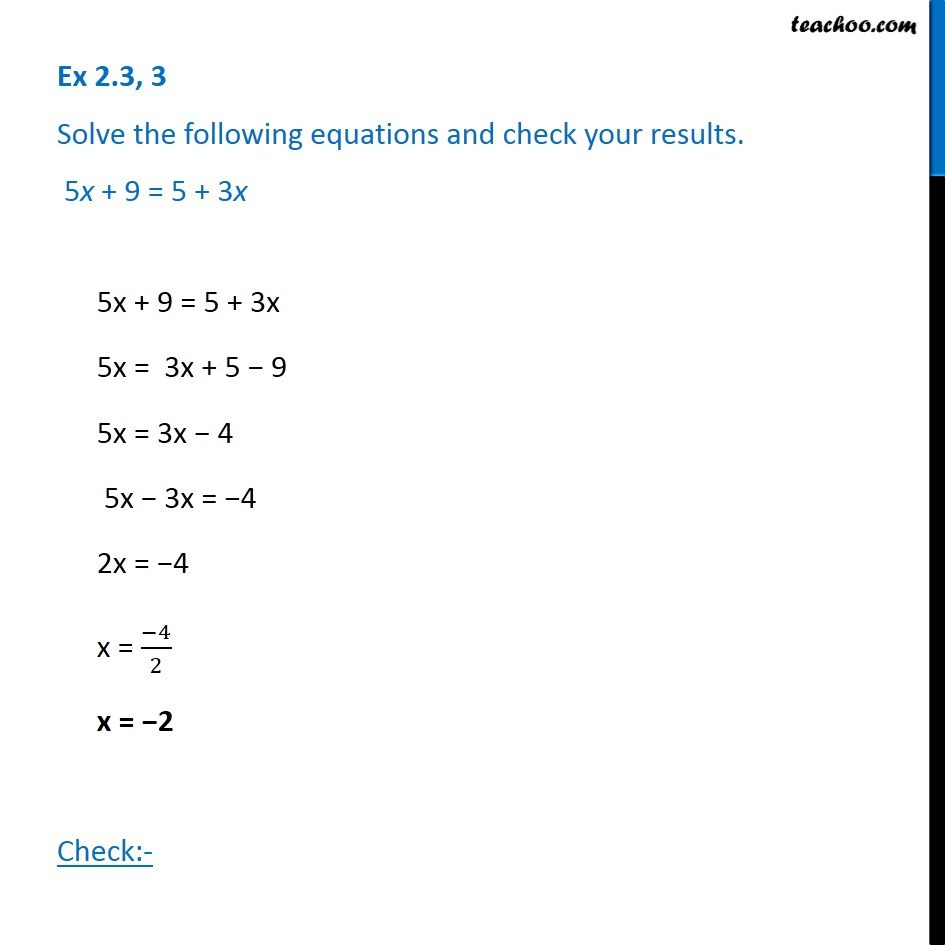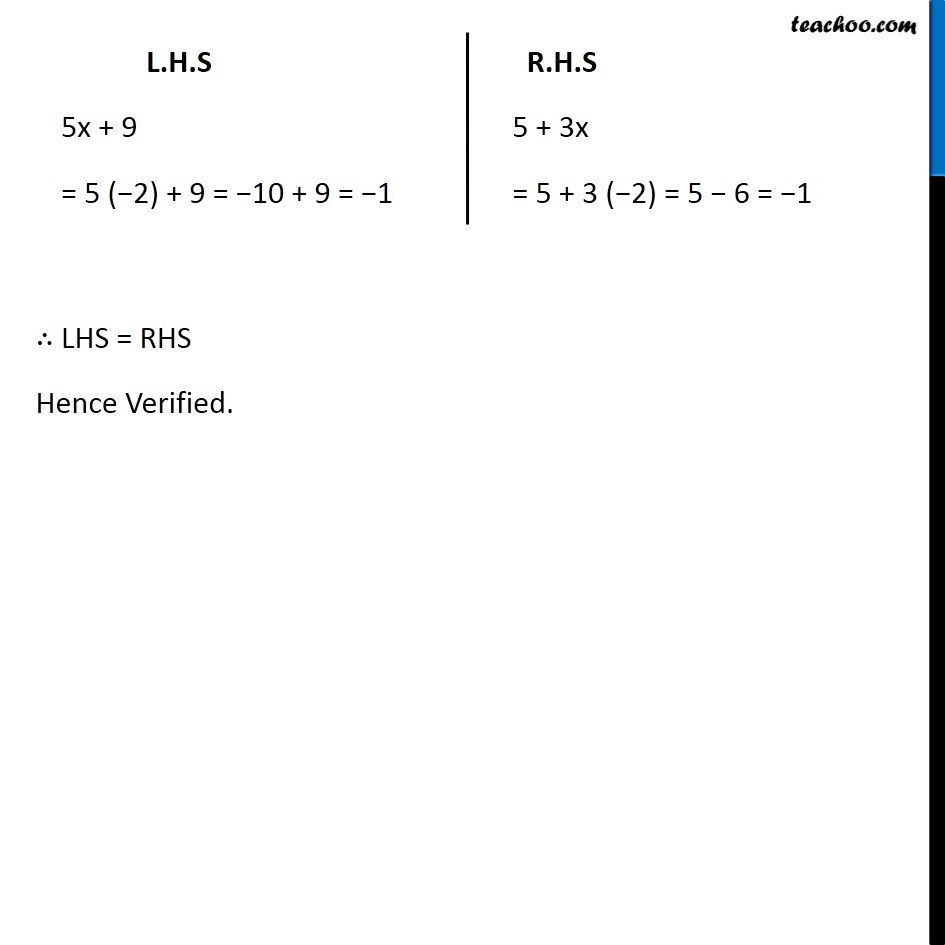Ex 2.3

Chapter 2 Class 8 Linear Equations in One Variable
Serial order wiseGet live Maths 1-on-1 Classs - Class 6 to 12

### Transcript

Ex 2.3, 3 Solve the following equations and check your results. 5x + 9 = 5 + 3x5x + 9 = 5 + 3x 5x = 3x + 5 − 9 5x = 3x − 4 5x − 3x = −4 2x = −4 x = (−4)/2 x = −2 Check:- L.H.S 5x + 9 = 5 (−2) + 9 = −10 + 9 = −1 R.H.S 5 + 3x = 5 + 3 (−2) = 5 − 6 = −1 ∴ LHS = RHS Hence Verified.